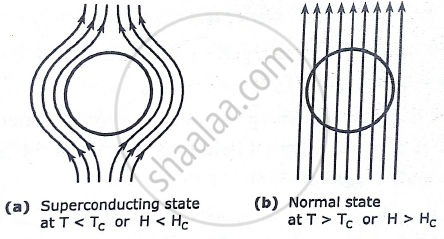# “Superconductor is a perfect diamagnetic”, Explain. - Applied Physics 1

“Superconductor is a perfect diamagnetic”, Explain.

#### Solution

A superconducting material kept in a magnetic field expels the magnetic flux out its body when cooled below the critical temperature and exhibits perfect diamagnetism. This is called MEISSNER EFFECT.

⦁ It is found that as the temperature of the specimen is lowered to Tc, the magnetic flux is suddenly and completely expelled from it. The flux expulsion continues for T < Tc. The
effect is reversible.

⦁ When the temperature is raised from below Tc. The flux density penetrates the specimen again at T = Tc and the material turns to the normal state.⦁ For the normal state the magnetic induction inside the specimen is given by:
B = μo (H+M) = μO(1+χ)H ………………………………………..(1)
Here H is the applied magnetic field , m is the magnetization produced within the specimen , is χ the susceptibility of the material and μo is the permeability of free space.
⦁ At T < T as seen above
B = 0
Hence equation (1) reduced to,

M = -H

And thus χ = M/H = -1

⦁ The specimen is therefore a perfect diamagnetic. The diamagnetism produces strong
repulsion to the external magnets.
⦁ This effect is used to identify a superconductor , in levitation effect and suspension effect.

Concept: Meissner Effect
Is there an error in this question or solution?